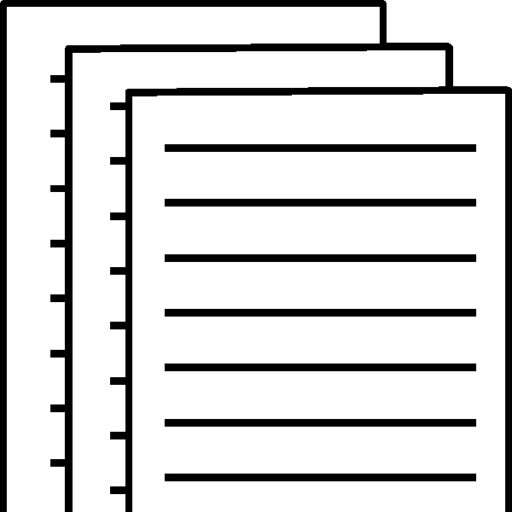## week 3 H-380-3-Homework

Problem 2-5A

The following are financial statements of Bramble Corp..

Net sales   \$2,231,500
Cost of goods sold   1,027,800
Interest expense   90,600
Income tax expense   70,170
Net income   \$ 124,330
Assets
Current assets
Cash   \$ 73,100
Debt investments   85,170
Accounts receivable (net)   189,700
Inventory   164,200
Total current assets   512,170
Plant assets (net)   590,700
Total assets   \$ 1,102,870
Liabilities and Stockholders’ Equity
Current liabilities
Accounts payable   \$ 173,000
Income taxes payable   36,670
Total current liabilities   209,670
Bonds payable   219,900
Total liabilities   429,570
Stockholders’ equity
Common stock   365,400
Retained earnings   307,900
Total stockholders’ equity   673,300
Total liabilities and stockholders’ equity   \$1,102,870

Additional information: The net cash provided by operating activities for 2017 was \$203,800. The cash used for capital expenditures was \$107,400. The cash used for dividends was \$32,170. The weighted-average number of shares outstanding during the year was 50,000.

(a) Compute the following values and ratios for 2017. (We provide the results from 2016 for comparative purposes.) (Round Current Ratio and Earnings per share to 2 decimal places, e.g. 15.25 and Debt to assets ratio to 1 decimal place, e.g. 78.9%. Enter negative amounts using either a negative sign preceding the number e.g. -45 or parentheses e.g. (45).)
(i) Working capital. (2016: \$160,500) Current ratio. (2016: 1.65:1) Free cash flow. (2016: \$48,700) Debt to assets ratio. (2016: 31%) Earnings per share. (2016: \$3.15)
(i) (ii) (iii) Working capital \$ Current ratio :1 Free cash flow \$ Debt to assets ratio % Earnings per share \$

Exercise 6-12

The following information is available for Pharoah Company for three recent fiscal years.

 Inventory Net sales Cost of goods sold 2017 2016 2015 \$552,440 \$569,374 \$337,484 1,955,006 1,692,420 1,322,224 1,532,544 1,277,699 936,594

Calculate the inventory turnover, days in inventory, and gross profit rate for 2017 and 2016. (Round inventory turnover to 1 decimal place, e.g. 5.2, days in inventory to 0 decimal places, e.g. 125 and gross profit rate to 1 decimal place, e.g. 5.2%.)

Inventory Turnover Days in Inventory 2017 2016 times times days days % %

### Exercise 8-14 (Part Level Submission)

Suppose the following information was taken from the 2017 financial statements of FedEx Corporation, a major global transportation/delivery company.

(in millions) Accounts receivable (gross) 2017 2016 \$ 3,408 \$ 4,611 3,144 4,284 264 327 32,081 35,794 6,739 6,864

Calculate the accounts receivable turnover and the average collection period for 2017 for FedEx Corporation. (Round answers to 1 decimal place, e.g. 12.5. Use 365 days for calculation.)

Accounts receivable turnover times days

#### (b)

Is accounts receivable a material component of the company’s total current assets?

Exercise 9-17

Crane Company reports the following information (in millions) during a recent year: net sales, \$10,185.0; net earnings, \$250.0; total assets, ending, \$5,130.0; and total assets, beginning, \$5,150.0.

(a) Calculate the (1) return on assets, (2) asset turnover, and (3) profit margin. (Round answers to 1 decimal place, e.g. 6.2% and 6.2.)

1. 2. Return on assets % Asset turnover times Profit margin %

To view and download a complete answer, scroll down to the bottom to pay